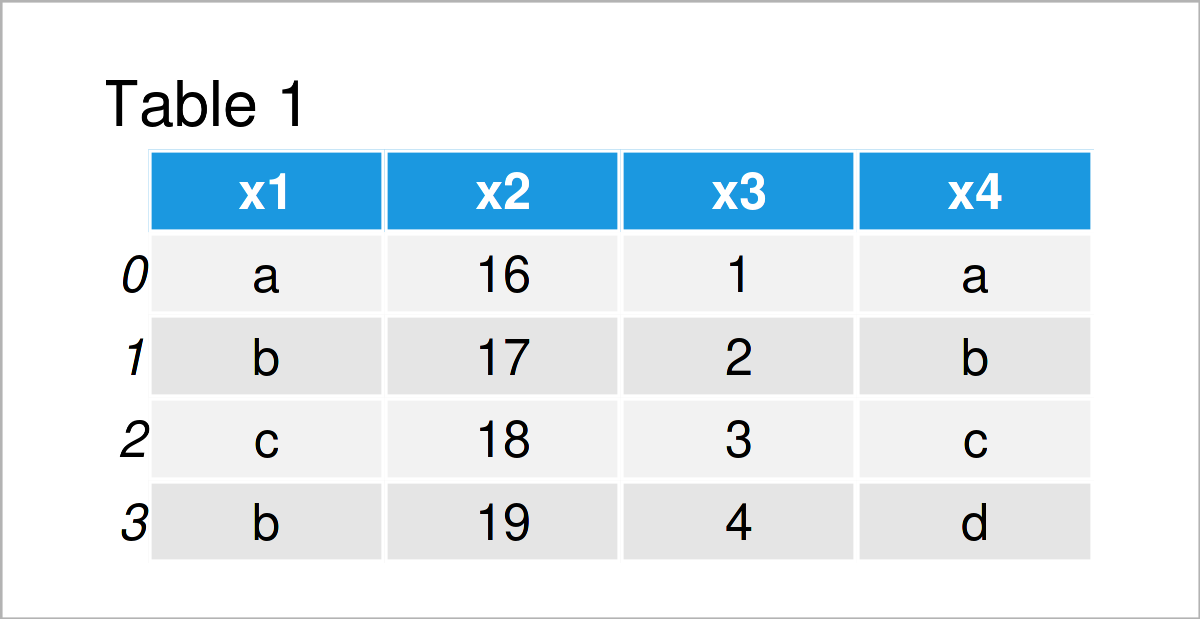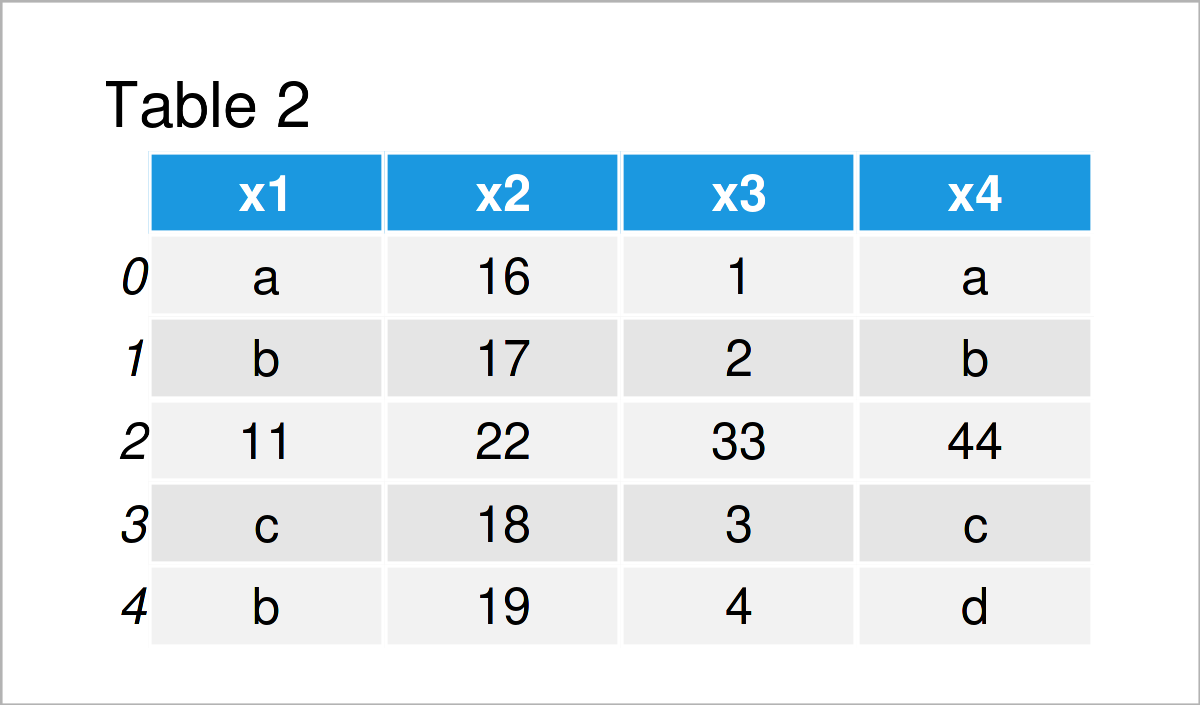# Insert Row at Specific Position of pandas DataFrame in Python (Example)

In this Python article you’ll learn how to insert a new row at an arbitrary position of a pandas DataFrame.

Let’s dive into it:

## Example Data & Libraries

First, we need to import the pandas library:

`import pandas as pd                                        # Load pandas library`

The pandas DataFrame below is used as basement for this Python programming tutorial:

```my_data = pd.DataFrame({"x1":["a", "b", "c", "b"],         # Create pandas DataFrame
"x2":range(16, 20),
"x3":range(1, 5),
"x4":["a", "b", "c", "d"]})
print(my_data)                                             # Print pandas DataFrame```Table 1 shows that our exemplifying data is composed of four rows and four variables.

Next, we need to create a list object containing the values that we want to insert as a new row in between our DataFrame:

```my_row = [11, 22, 33, 44]                                  # Create list
print(my_row)                                              # Print list
# [11, 22, 33, 44]```

Note that it’s important that this list has the same length as the number of columns of our DataFrame.

## Example: Add Row at Arbitrary Location of pandas DataFrame

In this example, I’ll demonstrate how to insert a new row at a particular index position of a pandas DataFrame.

For this task, we can use the loc attribute as well as the sort_index and reset_index functions as shown below:

```data_new = my_data.copy()                                  # Create copy of DataFrame
data_new.loc[1.5] = my_row                                 # Append list at the bottom
data_new = data_new.sort_index().reset_index(drop = True)  # Reorder DataFrame
print(data_new)                                            # Print updated DataFrame```As shown in Table 2, the previous syntax has created a new pandas DataFrame representing a combined version of our input DataFrame and list.

As you can see, the list has been added at the index position No. 2, i.e. some part of the DataFrame have been stacked on top of the list, and other parts of the DataFrame have been merged at the bottom of the list.

Note that we have reset the indices of our DataFrame using the reset_index function. This step is optional and only needs to be applied in case we want to have indices with consecutive integers.

## Video & Further Resources

Would you like to know more about the addition of a new row at a specific location of a pandas data set? Then I recommend watching the following video on my YouTube channel. I demonstrate the contents of this tutorial in the video:

Please accept YouTube cookies to play this video. By accepting you will be accessing content from YouTube, a service provided by an external third party.If you accept this notice, your choice will be saved and the page will refresh.

Furthermore, you could have a look at the related articles that I have published on www.statisticsglobe.com. I have published several tutorials on the concatenation of different data sources already: